# Optics

## Properties of Light and the Types of Images

WHAT IS WAVE?

Waves can be defines as the vibrations that include perturbation with a periodic spatial pattern and that pattern propagates in space. Waves will have a wavelength. Wave has the property of interference. Example: Sound waves when propagates produces crest and troughs.

CAN LIGHT BE CONSIDERED AS A WAVE?

To understand this we need to find the analogy between the characteristics of sound and light. When a crest and a trough meet in the trajectory, the two waves actually cancel each other. Similarly, when two sources emitting light waves are coordinated it also produces a pattern of alternating bright and dark bands on a screen. This experiment was done by Thomas Young to prove the wave pattern of light.

PROPERTIES OF LIGHT

There are two ways by which light propagates. These are

• i. Reflection

• ii. Refraction

• Christian Huygens was the one who proposed a mechanism for the propagation of light, called Huygens’ Principle: All points on a wavefront are sources of new waves, and the envelope of these secondary waves forms the new wavefront. Huygens’ Principle states a very fundamental property of waves, which clearly explains the phenomena of refraction. This chapter focuses mainly on refraction property.

REFRACTION OF LIGHT RAYS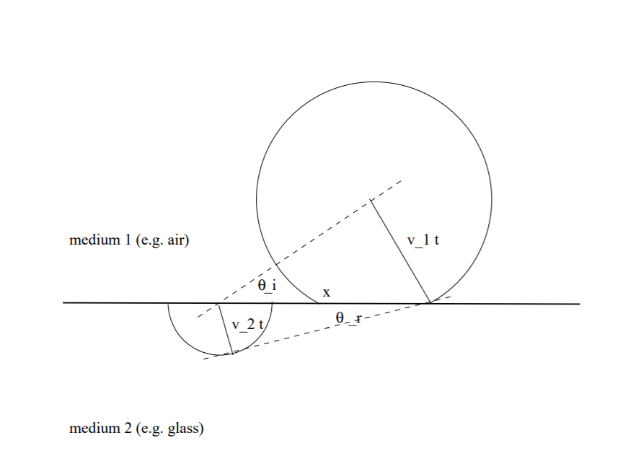Light propagating through a transparent material medium has its speed generally less than the speed in vacuum c. Now it was observed that a light ray changes its direction while passing from one medium to another. This phenomenon is known as refraction. When a plane wavefront approaches the interface between two media, a new wavefront propagates outwards at one end reaching the interface in a time t according to Huygens’ principle. On the other end, a new wavefront propagates into medium 2 more slowly, so that in the same time t it makes radius v2t. Now considering the angle of incidence θi and the angle of refraction θr between the incident wavefront and the interface, and between the refracted wavefront and the interface.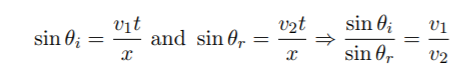Then the refractive index is defined as: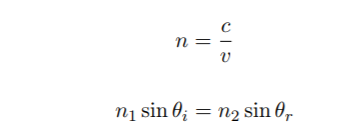This is called Snell's Law.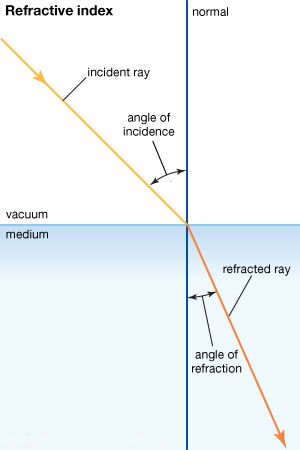Refractive indices are always greater than 1 except vacuum which has an index of 1. It is 1.33 for water. the refractive index may be associated with the density of the material, but that is not always true. It is better to consider optical density for calculating it.

IMAGES

an image is created from an object using an optical system. For example, a slide projector creates an
image of a slide on a screen. An extended object is treated as a collection of multiple point sources of light.

Images are of two types:

• 1. Real Images

• 2. Virtual Images

• Real Images

a real image is an image is said to be formed when it is located in the plane of convergence for the light rays originating from a given object. If a screen is placed in the plane of a real image the image will be visible on the screen. Examples of real images include the image seen projected on a cinema screen with the source being the projector or the image formed on a detector in the rear of a camera, and the image formation in the retina of the human eye. A real image is said to be formed when the rays converge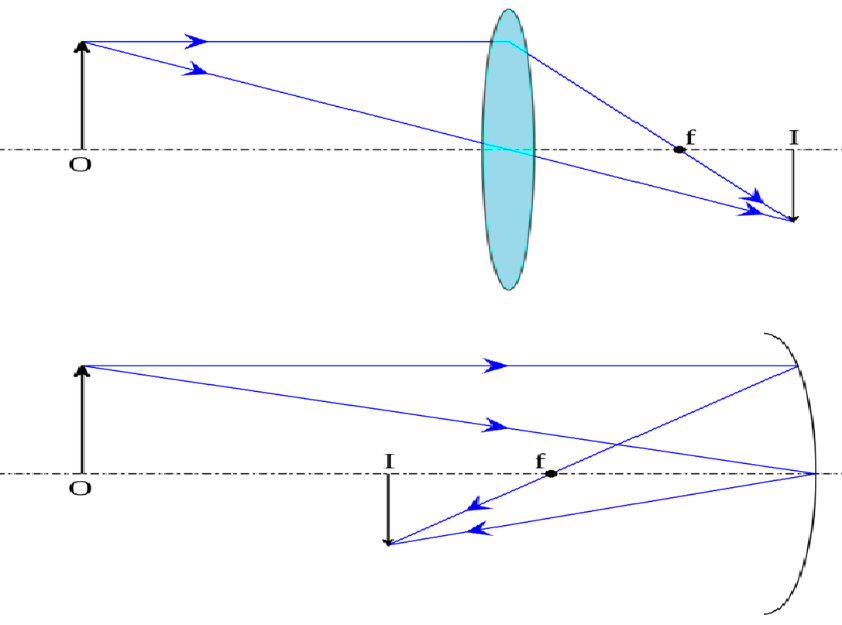The real image formed using a convex lens

Virtual Images

a virtual image is an image formed when the outgoing rays from a point on an object get diverged or they just appear to converge from a point only. The image seems to be located at the point of apparent divergence/convergence as the rays never really converge. Thus, a virtual image can never be projected onto a screen. In diagrams of optical systems, virtual rays are thus mostly represented by dotted lines. Virtual images can be located by tracing the real rays that emerge from an optical device (lens, mirror, or some combination) backward to a perceived point of origin.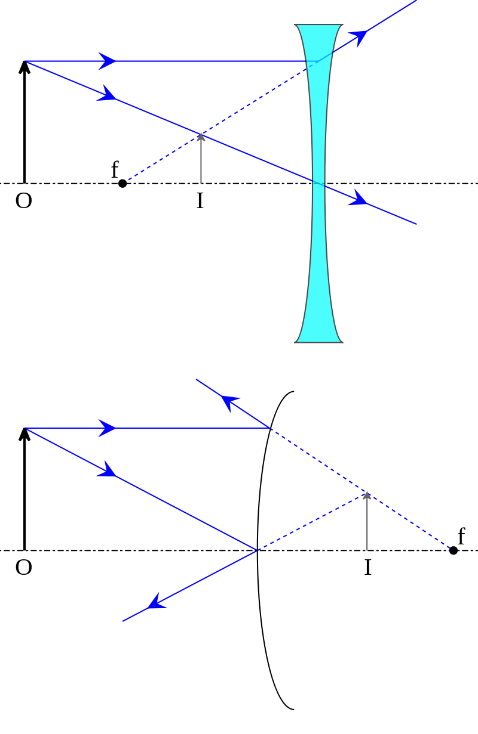The above diagram depicts the formation of a virtual image using a diverging lens.

THE MIRROR EQUATION

We can calculate the location of the image from the position of the object and of the mirror’s focal point with the help of the mirror equation. These positions are measured using the object distance p measured along the axis from the vertex of the mirror, where the axis is intersecting the mirror; the image distance i, and the focal length f.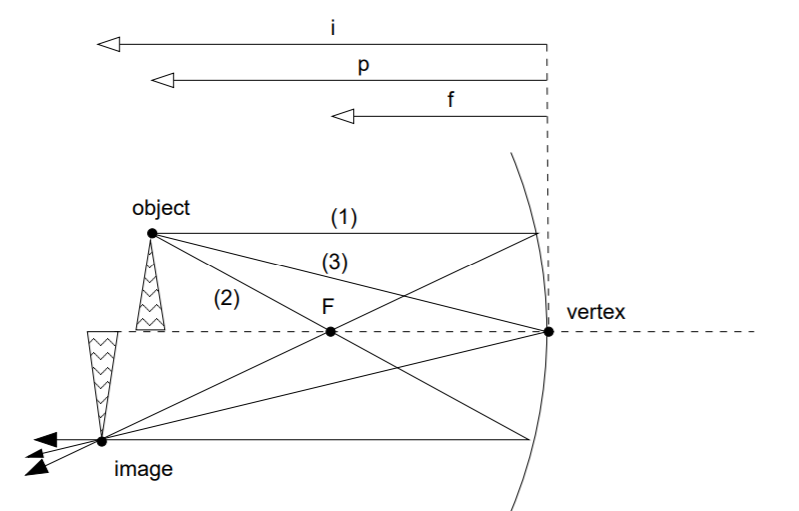The mirror equation assumes that the curvature of the mirror is extremely small, which is only true if the object is relatively small and quite close to the optical axis. In that case, we can consider the mirror approximately flat. The triangles 4OP F and 4F QI are similar then. This means that the following equation can be derived: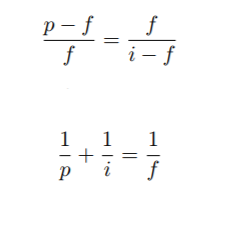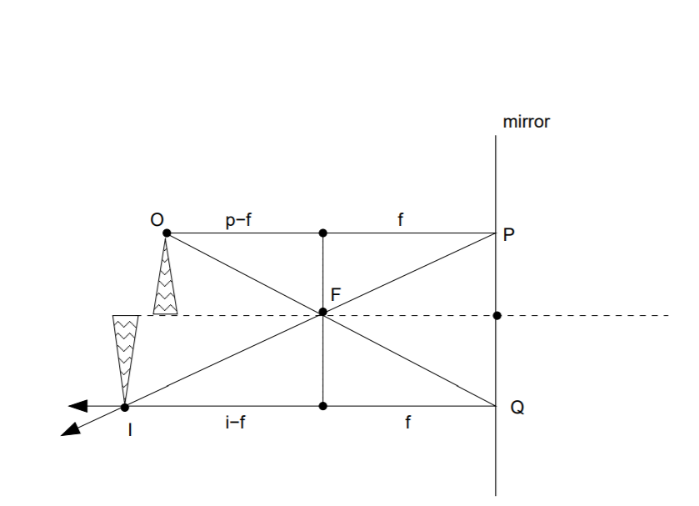LENS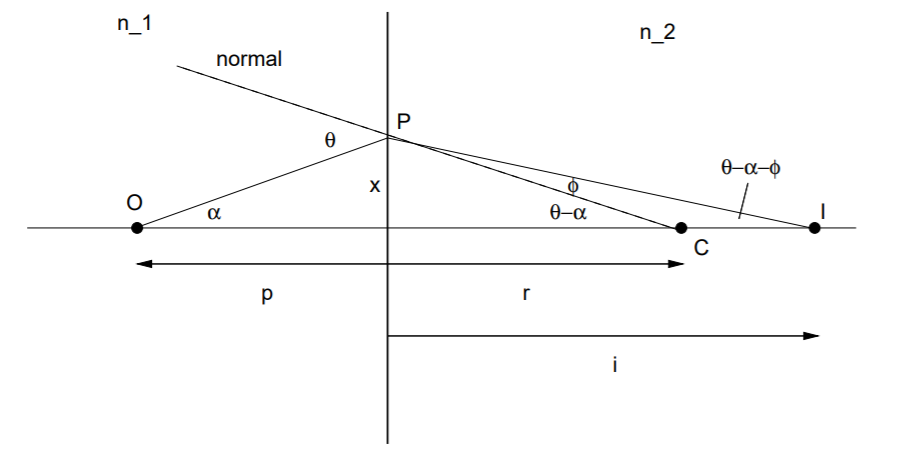In most of the cases discussed here, the curvature of the interface has been considered as very small, so that it can be considered as a flat surface. In the figure, a point object at O is emitting a ray of light along the optical axis, and another ray of light is getting refracted at the interface and intersecting the first one to form an image at I. Let the radius of curvature of the interface is r; as usual, the object distance to the interface be p and the image distance be i.

If the surface is convex and the center of curvature C is to the right. Then for the oblique ray, the incidence angle is θ and the refracted angle is φ. Then, as per the exterior angle theorem,
6 P CO = θ − α and 6 P IC = θ − α − φ.
In the small angle assumption, Snell’s law becomes
n1θ = n2φ
and the angles can be approximated as follows: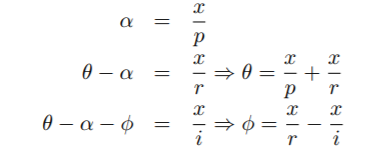and substituting θ and φ in Snell's law, we get after canceling x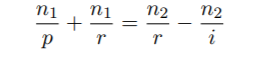which can be rearranged more meaningfully to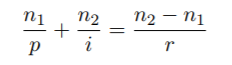If the light is passing from the air of refractive index n1 = 1 to a glass of index n2 = n, then the eq is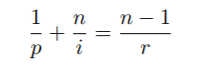Locating the image

In a lens, two consecutive refractions happen. Those are from air to glass, and then from the glass back into the air. Then applying the lens equation we can write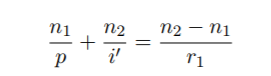here r1 is the curvature radius for the first surface. The image formed after the first refraction becomes the object of the second refraction, and its distance from the second surface is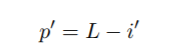so that the final image formed at a distance i from the second surface is given by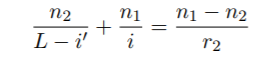In the thin lens approximation, L → 0 so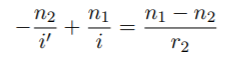Eliminating the intermediate image we can arrive at a single relation between object and image distances, adding the two equations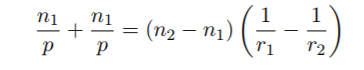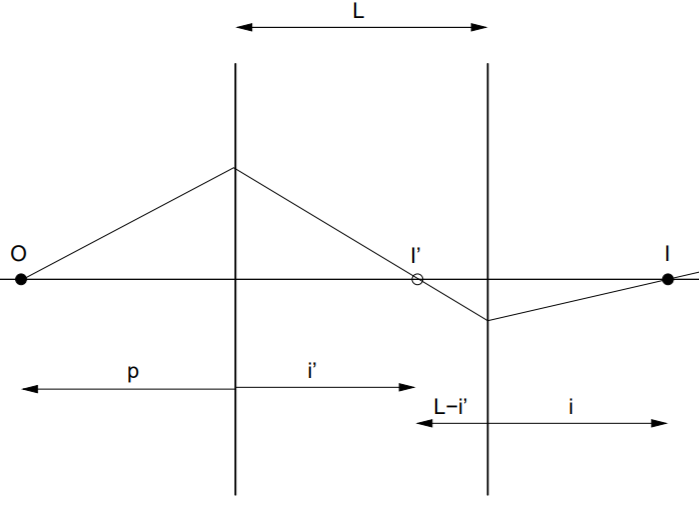The lens maker equation

In most of the cases, the outside medium is air – n1 = 1–, and the material of the lens is glass, with a refractive index n2 = n though it depends on the particular type of glass used. In this case, the equation will be: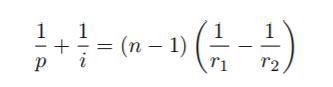It is important to notice that the right-hand side is dependent on the characteristics of the lens: material it is made of, and the curvature radii of its surfaces. Let us assume that it has dimensions of (length)−1; and when the object is at infinity, making the incident rays are parallel to the axis, the image is formed at a distance from the lens which is equal to the inverse of the right-hand side. All this indicates that a focal length for the lens can be calculated in the following way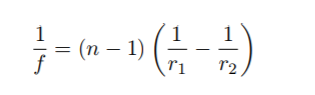This is known as the lensmaker’s equation. It helps a lensmaker to determine what curvature radii he should achieve while grinding a lens to obtain a desired focal length f, under the condition that he’s working with a particular type of glass of refractive index n.

Converging and diverging lens

With the positive focal length, the image of an object at infinity is formed by rays that are converging at a point behind the lens. Such a lens is known as the converging. On the other hand, in case of a negative focal length, the rays from an object at infinity get diverged after passing through the lens, thus they appear to come from a point somewhere in front of the lens. This is known as a diverging lens.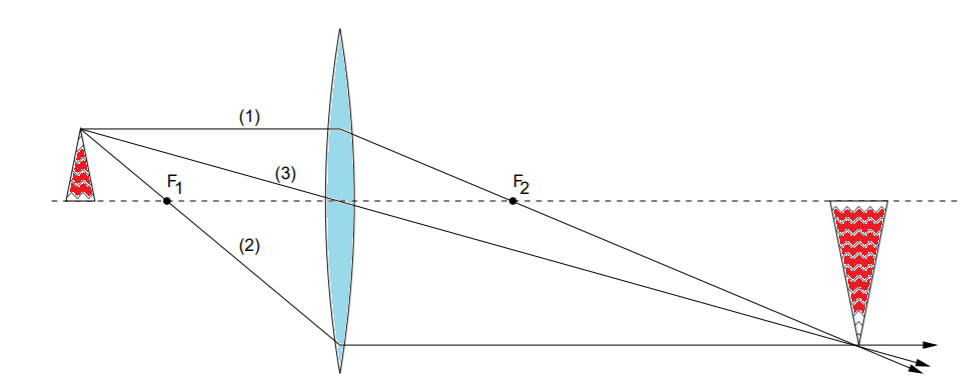Converging lens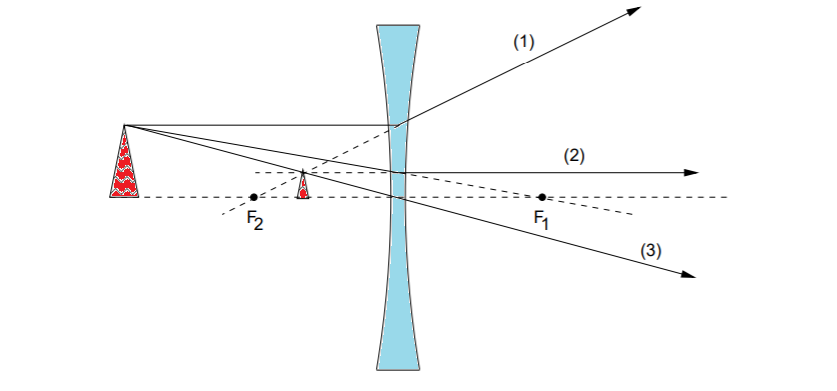Diverging Lens

The image was formed at the point of intersection not of the light rays emerging from the lens, but of their extension behind the lens that is at the backward. This results in the formation of a virtual image: Such images can never be projected on a screen. In fact, since the image is formed behind the lens, so even if a screen is placed there, the light would get blocked and would not be able to pass through the lens at all.

IMPLEMENTATION OF LENS

Various combinations of the lens are used in manufacturing various optical devices. Some of those devices include magnifying glass, telescope, camera and many more.